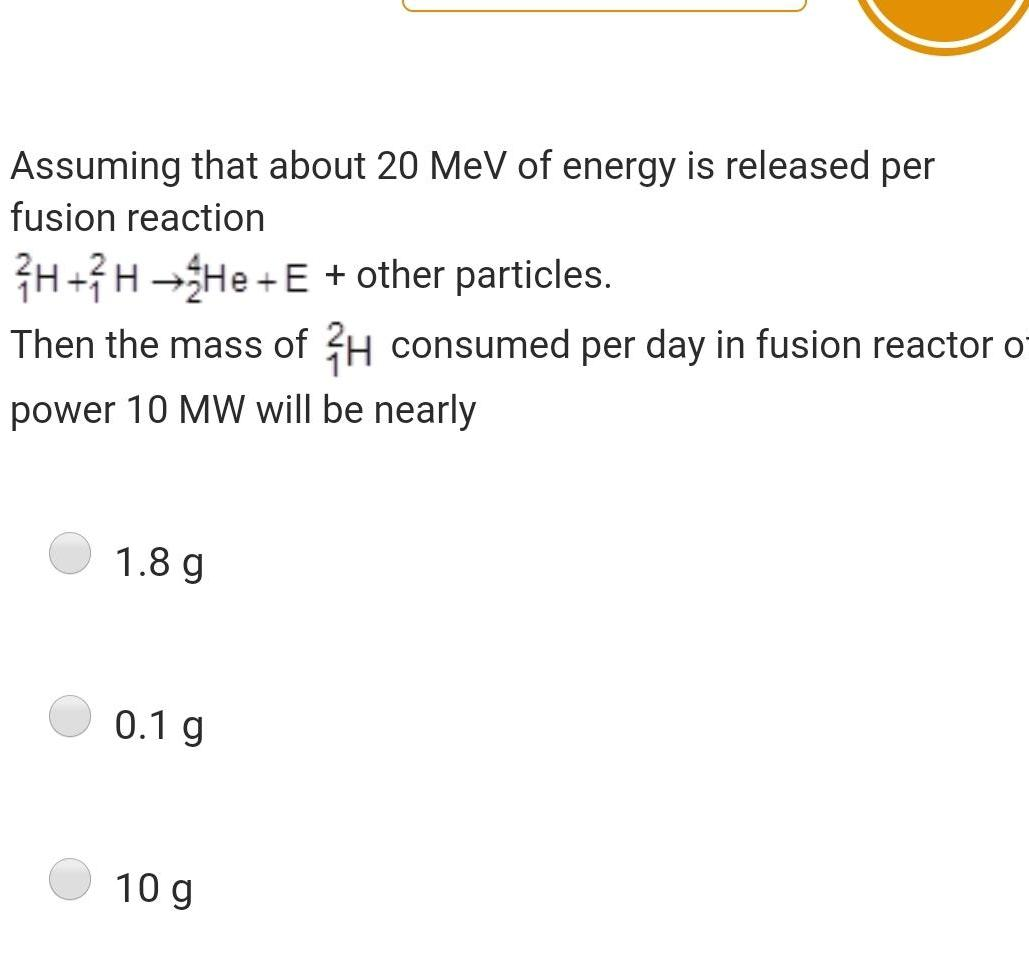Physics
Nucleus
Assuming that about 20 MeV of energy is released per fusion reaction H H He E other particles Then the mass of H consumed per day in fusion reactor of power 10 MW will be nearly 1 8 g 0 1 g 10 g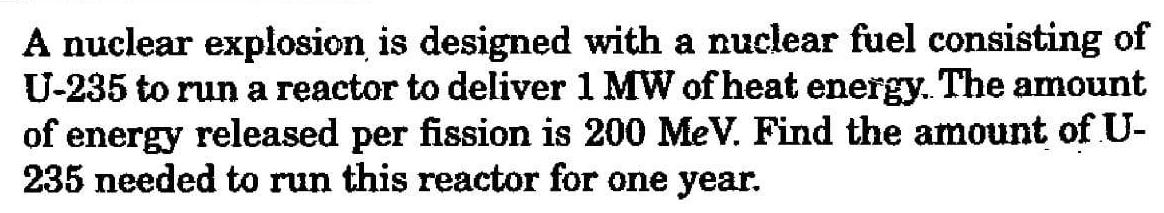Physics
Nucleus
A nuclear explosion is designed with a nuclear fuel consisting of U 235 to run a reactor to deliver 1 MW of heat energy The amount of energy released per fission is 200 MeV Find the amount of U 235 needed to run this reactor for one year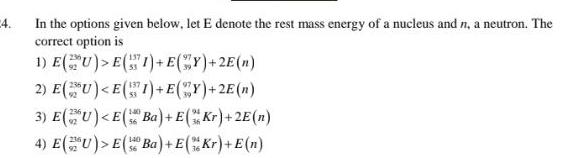Physics
Nucleus
4 In the options given below let E denote the rest mass energy of a nucleus and n a neutron The correct option is 1 E U E I E Y 2E n 2 E U E 1 E Y 2E n 3 E U E Ba E Kr 2E n E U E Ba E Kr E n 4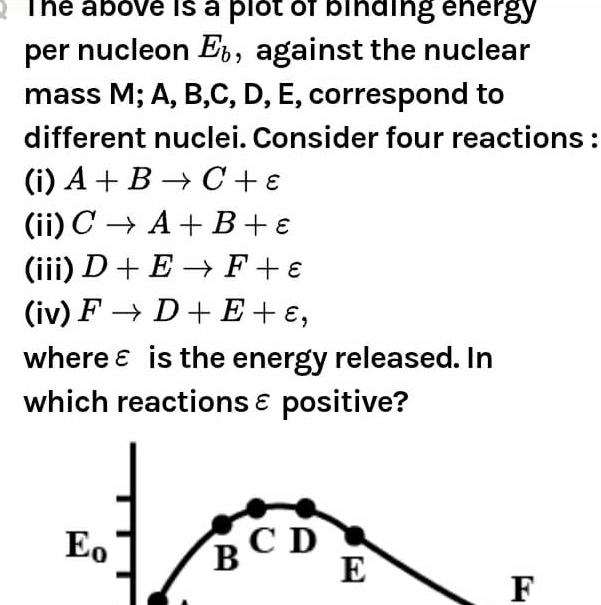Physics
Nucleus
The above is a plot of binding energy per nucleon Eb against the nuclear mass M A B C D E correspond to different nuclei Consider four reactions i A B C ii C A B iii D E F iv F D E where is the energy released In which reactions positive Eo BCD E F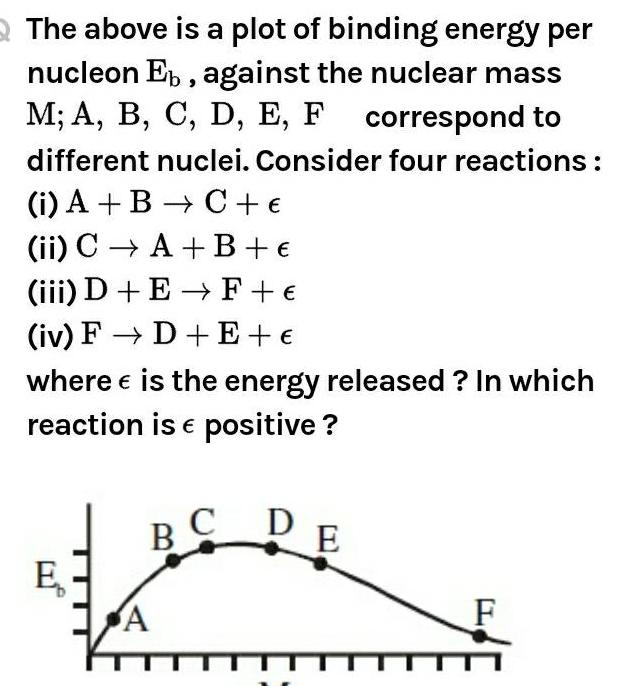Physics
Nucleus
The above is a plot of binding energy per nucleon Eb against the nuclear mass M A B C D E F correspond to different nuclei Consider four reactions i A B C ii C A B iii D E F iv F D E where is the energy released In which reaction is positive E BC A D E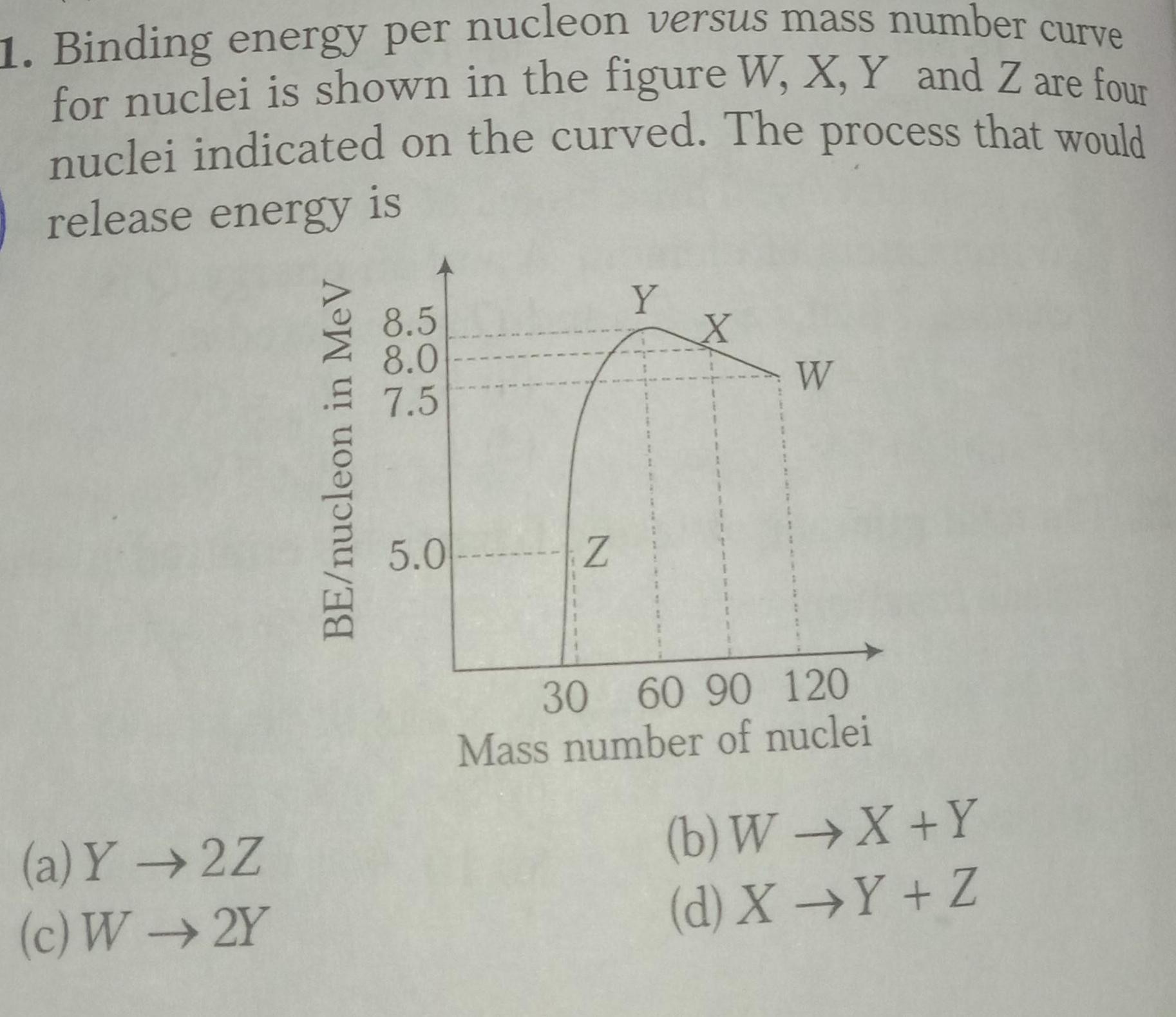Physics
Nucleus
1 Binding energy per nucleon versus mass number curve for nuclei is shown in the figure W X Y and Z are four nuclei indicated on the curved The process that would release energy is a Y 2Z c W2Y BE nucleon in MeV 8 5 8 0 7 5 5 0 Z Y X W 30 60 90 120 Mass number of nuclei b W X Y d X Y Z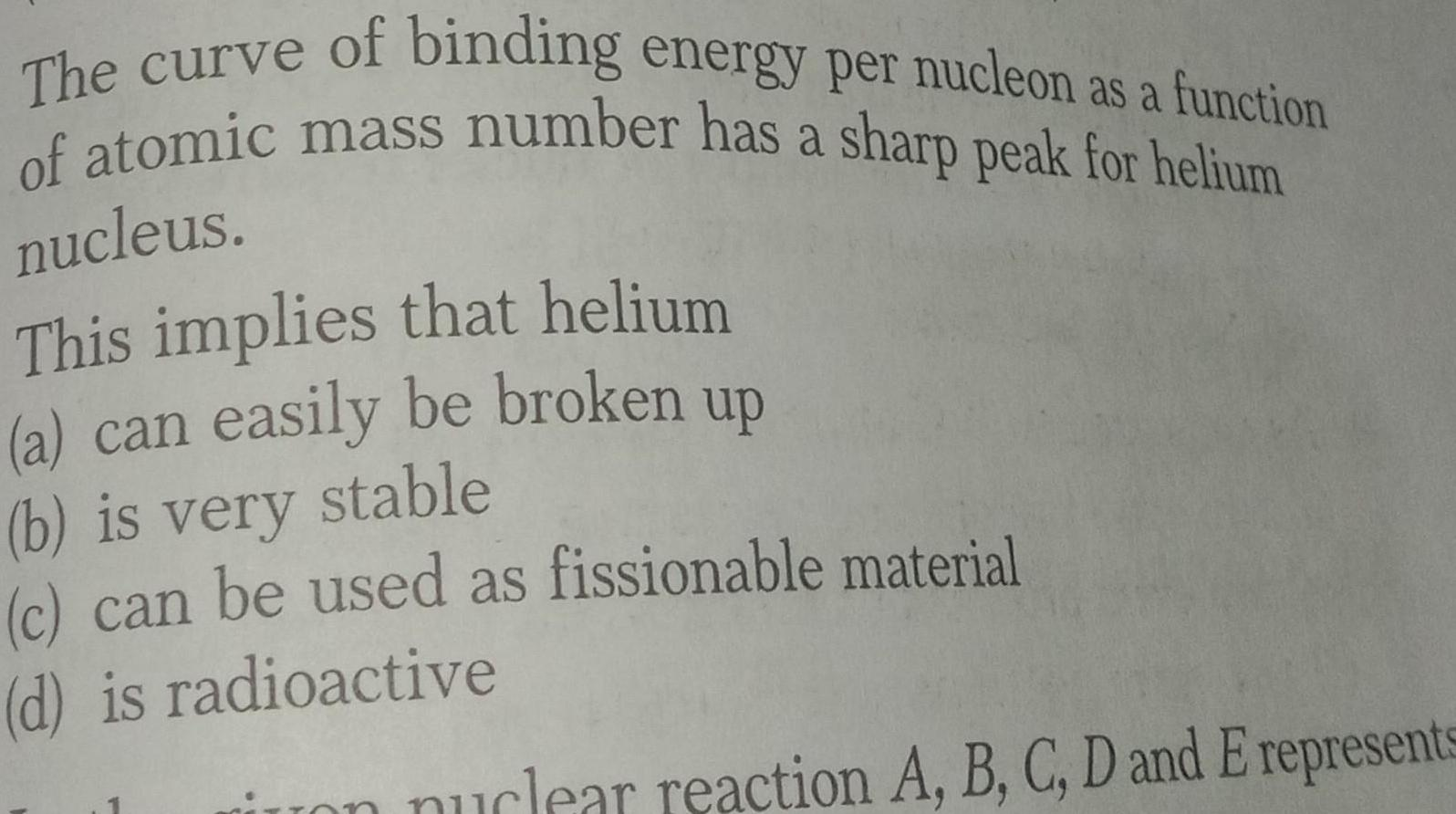Physics
Nucleus
The curve of binding energy per nucleon as a function of atomic mass number has a sharp peak for helium nucleus This implies that helium a can easily be broken up b is very stable c can be used as fissionable material d is radioactive on nuclear reaction A B C D and E represents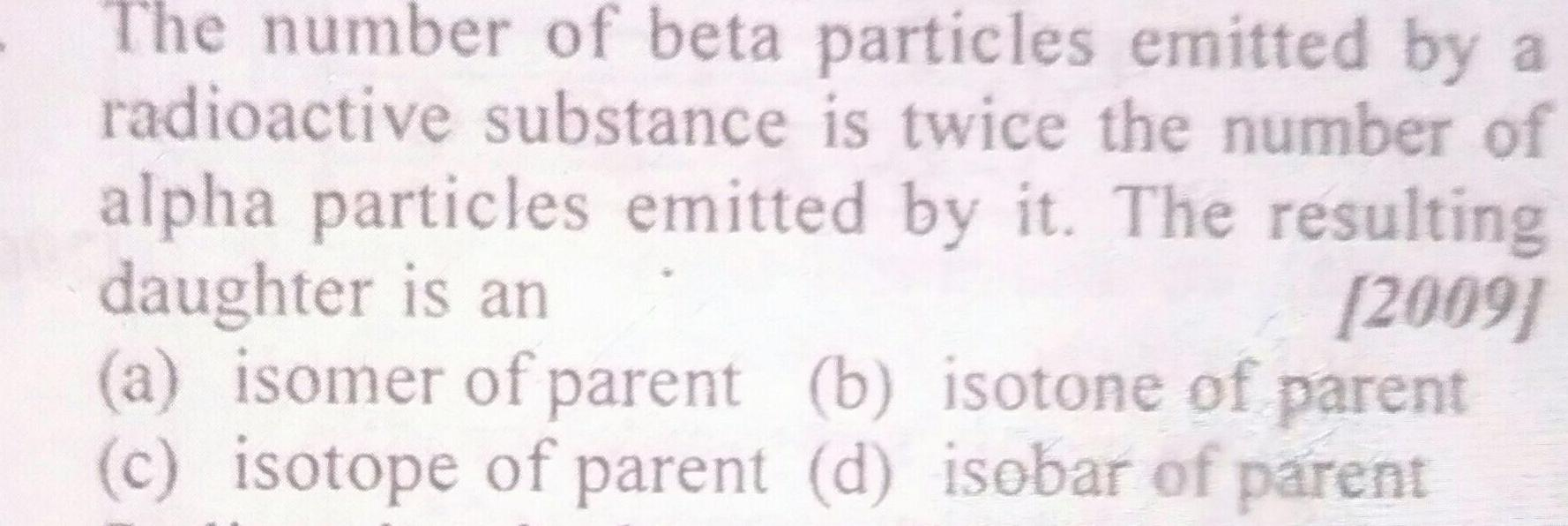Physics
Nucleus
The number of beta particles emitted by a radioactive substance is twice the number of alpha particles emitted by it The resulting daughter is an 2009 a isomer of parent b isotone of parent c isotope of parent d isobar of parent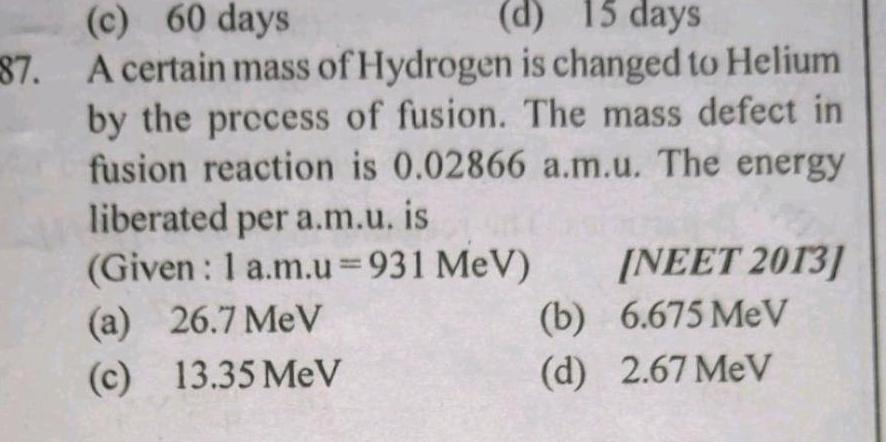Physics
Nucleus
c 60 days d 15 days 87 A certain mass of Hydrogen is changed to Helium by the process of fusion The mass defect in fusion reaction is 0 02866 a m u The energy liberated per a m u is Given 1 a m u 931 MeV a 26 7 MeV c 13 35 MeV NEET 2013 6 675 MeV b d 2 67 MeV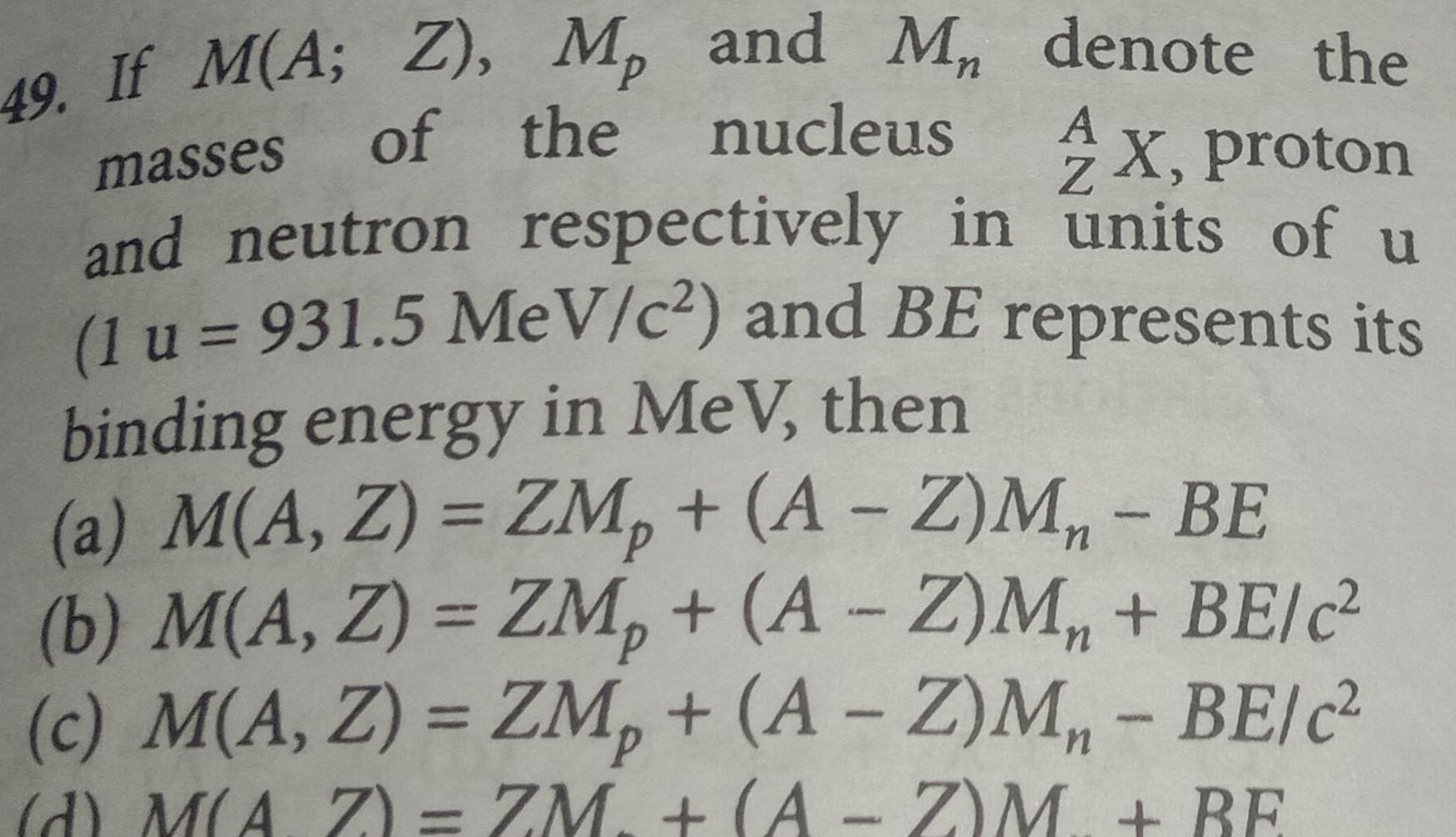Physics
Nucleus
49 If M A Z M and M denote the of the nucleus masses AX proton and neutron respectively in units of u 1 u 931 5 MeV c and BE represents its binding energy in MeV then a M A Z ZM A Z M BE b M A Z ZM A Z M BE c c M A Z ZM A Z M BE c d M AZ ZM A Z M BE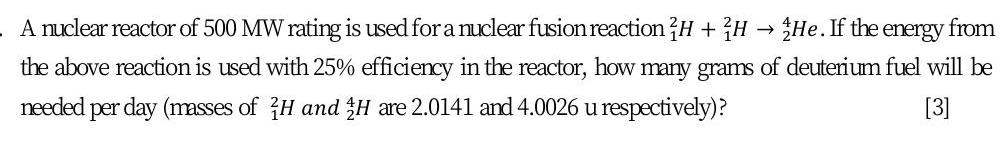Physics
Nucleus
A nuclear reactor of 500 MW rating is used for a nuclear fusion reaction 2H H He If the energy from the above reaction is used with 25 efficiency in the reactor how many grams of deuterium fuel will be needed I per day masses of H and H are 2 0141 and 4 0026 u respectively 3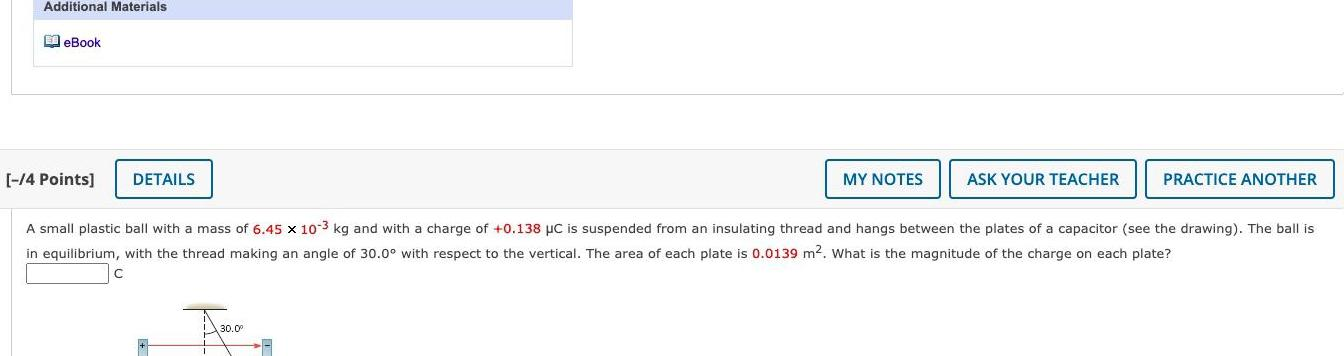Physics
Nucleus
Additional Materials EeBook 4 Points DETAILS MY NOTES 30 0 ASK YOUR TEACHER A small plastic ball with a mass of 6 45 x 10 3 kg and with a charge of 0 138 C is suspended from an insulating thread and hangs between the plates of a capacitor see the drawing The ball is in equilibrium with the thread making an angle of 30 0 with respect to the vertical The area of each plate is 0 0139 m What is the magnitude of the charge on each plate C PRACTICE ANOTHER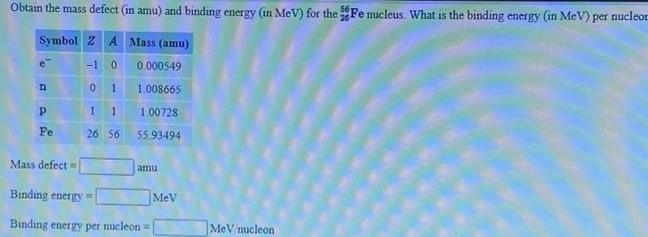Physics
Nucleus
Obtain the mass defect in amu and binding energy in MeV for the Fe nucleus What is the binding energy in MeV per nucleor Symbol Z A Mass amu 10 n P Fe 0 1 1 1 26 56 0 000549 1 008665 1 00728 5 93494 Mass defect Binding energy Binding energy per nucleon amu MeV MeV nucleon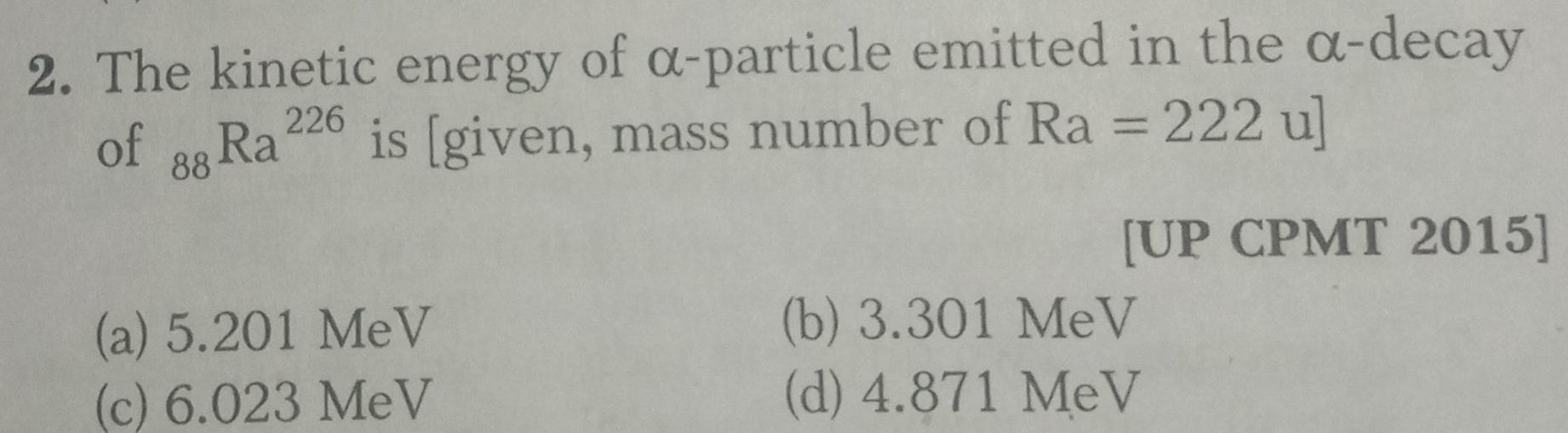Physics
Nucleus
2 The kinetic energy of a particle emitted in the a decay of 88 Ra 226 is given mass number of Ra 222 u a 5 201 MeV c 6 023 MeV UP CPMT 2015 b 3 301 MeV d 4 871 MeV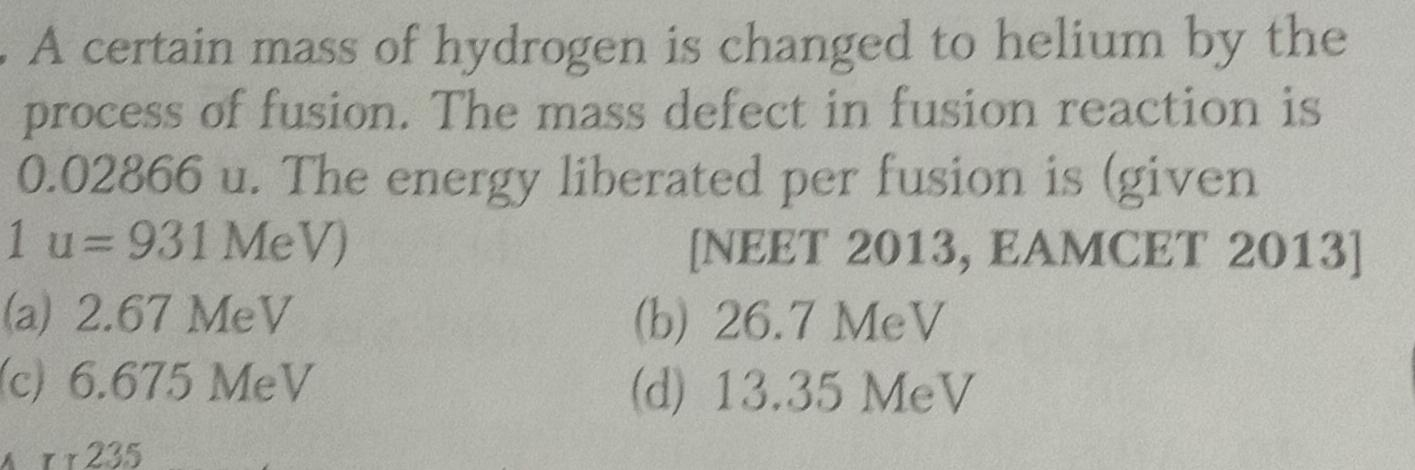Physics
Nucleus
A certain mass of hydrogen is changed to helium by the process of fusion The mass defect in fusion reaction is 0 02866 u The energy liberated per fusion is given 1 u 931 MeV a 2 67 MeV c 6 675 MeV A TT235 NEET 2013 EAMCET 2013 b 26 7 MeV d 13 35 MeV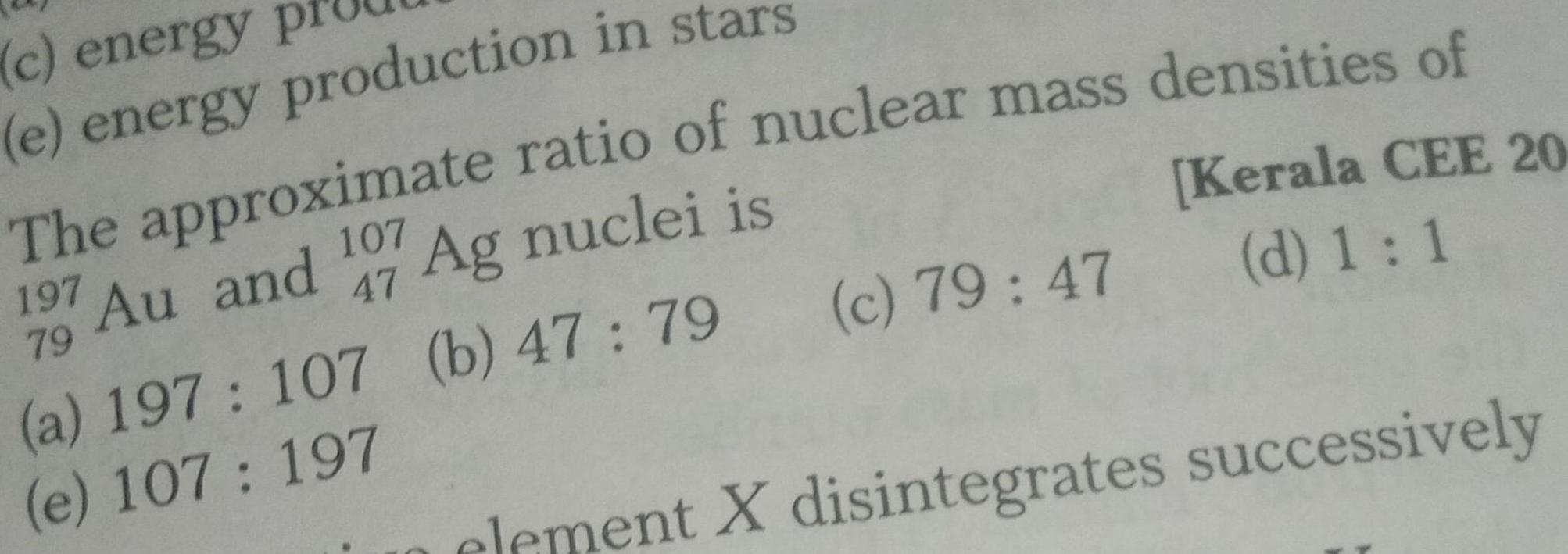Physics
Nucleus
c energy e energy production in stars Kerala CEE 20 d 1 1 The approximate ratio of nuclear mass densities of 197 Au and 107 Ag nuclei is 47 79 a 197 107 b 47 79 c 79 47 e 107 197 element X disintegrates successively